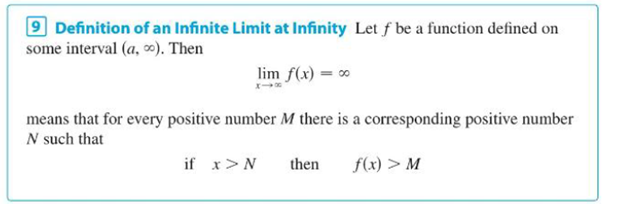Chapter 2.6, Problem 78E### Single Variable Calculus: Early Tr...

8th Edition
James Stewart
ISBN: 9781305270343

#### Solutions

Chapter
Section### Single Variable Calculus: Early Tr...

8th Edition
James Stewart
ISBN: 9781305270343
Textbook Problem

# Prove, using Definition 9, that lim x → ∞ x 3 = ∞ . Definition 9To determine

To prove: The value of limxx3=.

Explanation

Definition:

“Let f be a function defined on some interval (a,). Then limxf(x)= means that for every positive number M there is a corresponding positive number N such that if x>N then f(x)>M

Graph:

Use the rough graph of the function.

Proof:

For every M, x3>M.

M is a positive integer, M=N3.

N is a positive integer, x3>N3

x3N3>0

(x3N3=(xN)(x2+xN+N2)

### Still sussing out bartleby?

Check out a sample textbook solution.

See a sample solution

#### The Solution to Your Study Problems

Bartleby provides explanations to thousands of textbook problems written by our experts, many with advanced degrees!

Get Started

#### Expand each expression in Exercises 122. (3x+1)(2x2x+1)

Finite Mathematics and Applied Calculus (MindTap Course List)

#### 16. If , find the following. (a) (b) (c)

Mathematical Applications for the Management, Life, and Social Sciences

#### An integral for the solid obtained by rotating the region at the right about the x-axis is:

Study Guide for Stewart's Single Variable Calculus: Early Transcendentals, 8th